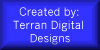# Cactus Programming Environment Tutorial

## Contents

### Introduction

Cactus is a brand new programming language created in June of 1999 by Kevin P. Albrecht. It is an interpreted language designed simply for recreation. Cactus files use the extension .cts and are written using the following procedures.

• Contents

### Procedure

Writing Cactus programs is very simple. You simply create an ascii text file with the folloing keywords of your choice, one per line. When using mathematical operators, the result will replace the value in the first <variable-name> you supply.

• Contents

### Keywords

`\$` <comment text>
Insert comments into a Cactus file.
`name` <program name
Indicate the name of the program.
`new`` <integer or decimal>` <variable-name>
Create a new variable initialized to zero.
`grab` <variable-name>
`print` <literal or variable-name>
Print the data.
`equ` <variable-name> <literal or variable-name>
Put the value of the second into the first.
`add` <variable-name> <literal or variable-name>
Add the value of the second to the first.
`sub` <variable-name> <literal or variable-name>
Subtract the value of the second from the first.
`mul` <variable-name> <literal or variable-name>
Multiply the value of the second to the first.
`div` <variable-name> <literal or variable-name>
Divide the value of the second from the first.
• Contents

### Examples

The following are some simple examples to help you get started programming with Cactus.

This program converts a celsius temperature into a fahrenheit temperature.

``` \$ Beginning of Cactus file. name ctof new decimal d grab d mul d 9.0 div d 5.0 add d 32.0 print d \$ End of Cactus file. ```

This program converts a fahrenheit temperature into a celsius temperature.

``` \$ Beginning of Cactus file. name ftoc new decimal d grab d sub d 32.0 mul d 5.0 div d 9.0 print d \$ End of Cactus file. ```
• Contents